【一猫汽车网西安站】随着飞机的缓缓降落，当我走出舱门那一刻。伴随着如同山水画卷的景象，我意识到已经身处于中国最北端的土地上，身处于这漫天大雪之中，身为北方人的我也难掩心中的兴奋和激动。我知道，很快我就可以在这大雪纷飞的土地上撒点野了，而陪伴我的就是新一代SUBARU XV。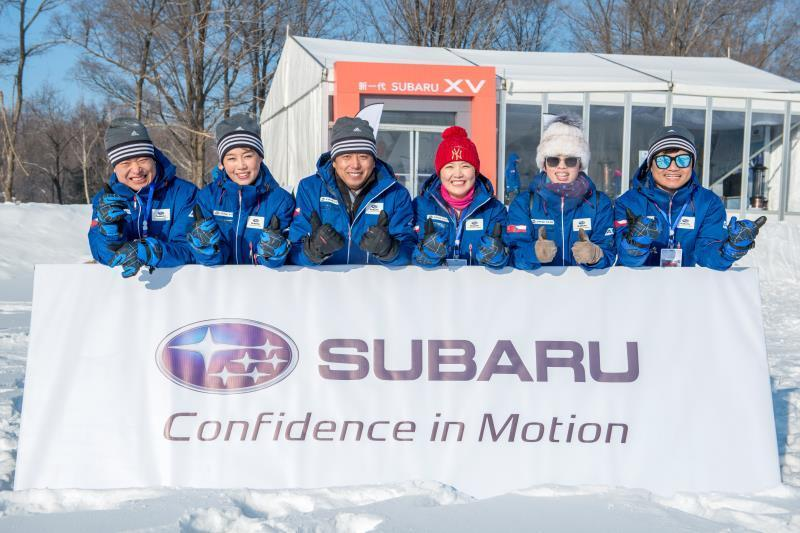“冬至”前后，太阳从东南方升起，西南方落下，太阳在地平之上的时间甚短，也就导致了高纬度地区的夜晚十分的漫长。经过“难熬”的一晚之后，终于迎来了本次活动的主题——新一代SUBARU XV华北媒体试驾会。会场上，一边是排列整齐的新款斯巴鲁全系车型和兴奋的媒体老师们，一边是安静而白雪皑皑的山林。阳光照射在车身上有着别样的美感。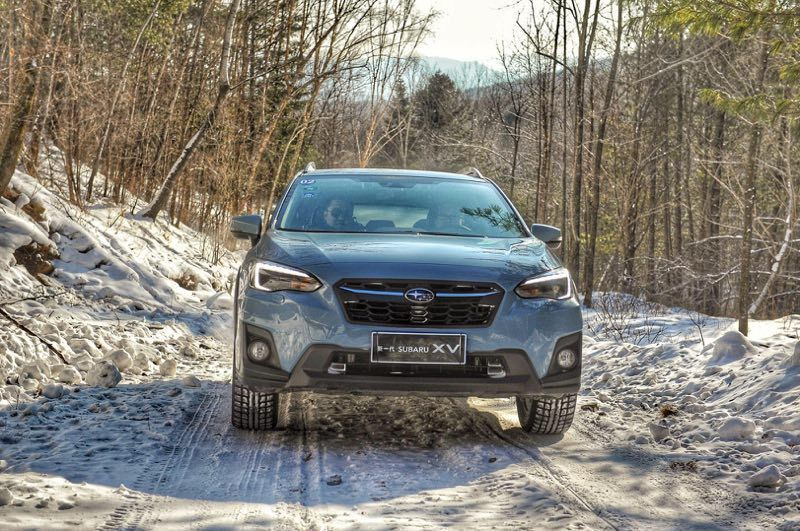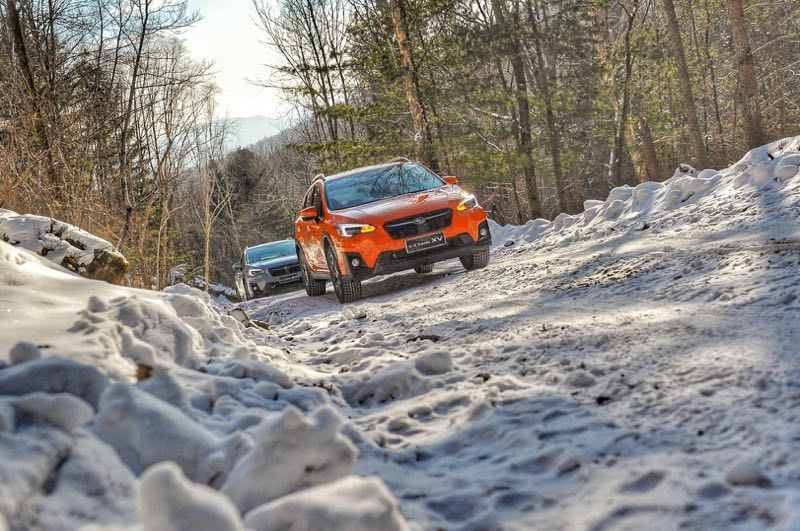接下來我想说说本次试驾会上的重点车型--新一代斯巴鲁XV。作为斯巴鲁新一代全球化平台SGP开发的首款引入中国市场的新车，斯巴鲁XV时隔5年迎来了它的首次换代。而扎实的用料和可靠的品质领斯巴鲁XV在中国市场上交出了一份满意的答卷。不就之前，XV也迎来的新一代换代产品的推出，更多的媒体将新一代XV看做是斯巴鲁翼豹的跨界产品，但是笔者认为，尽管身披跨界的外衣，新一代XV在骨子里仍旧是一款十分典型的斯巴鲁。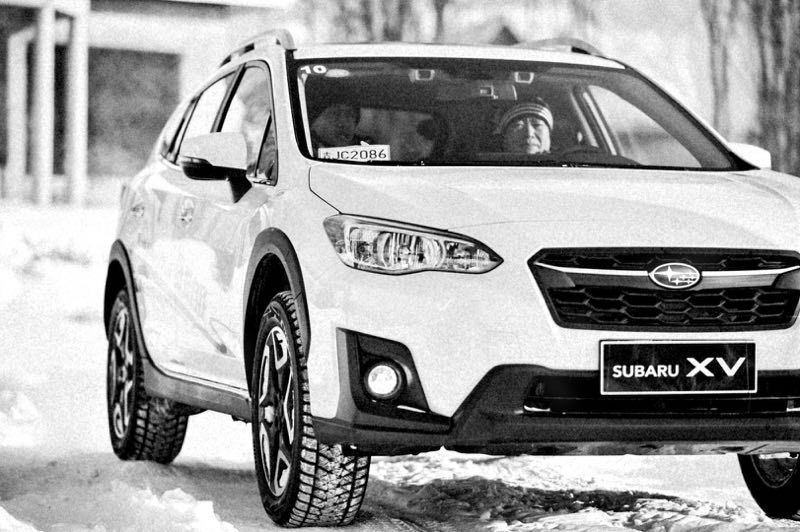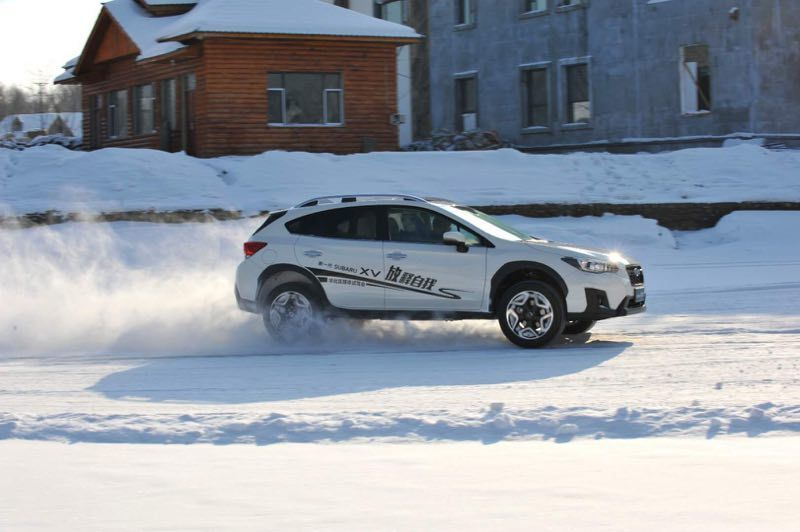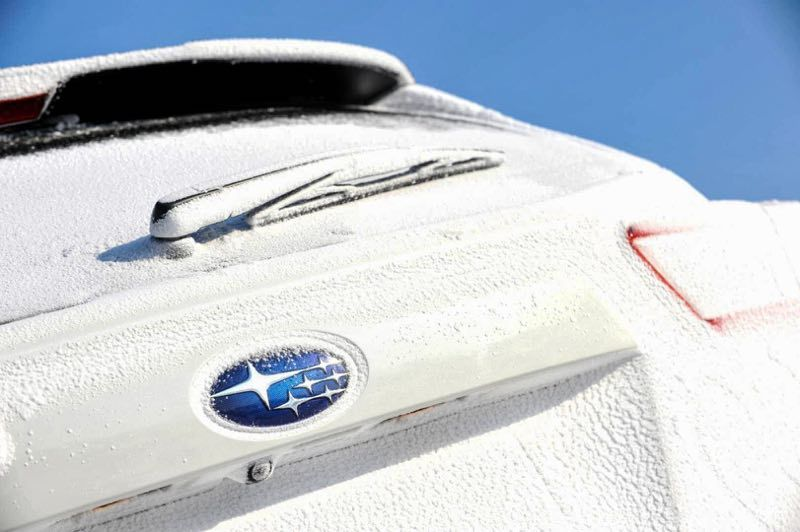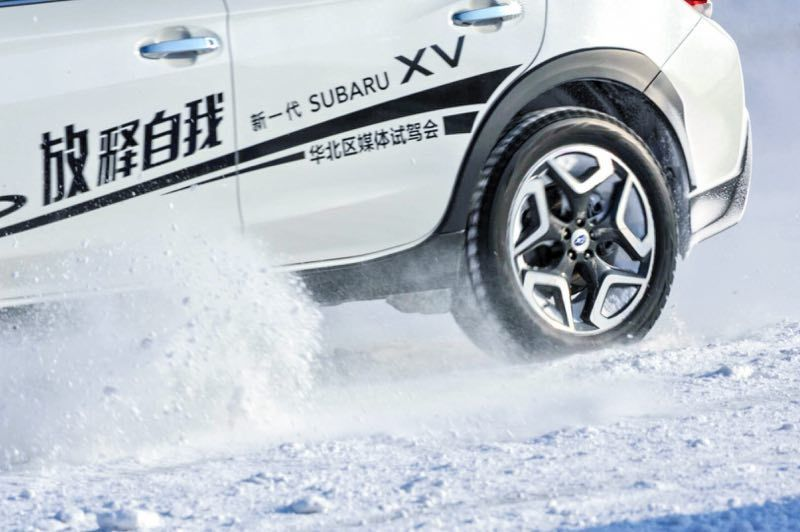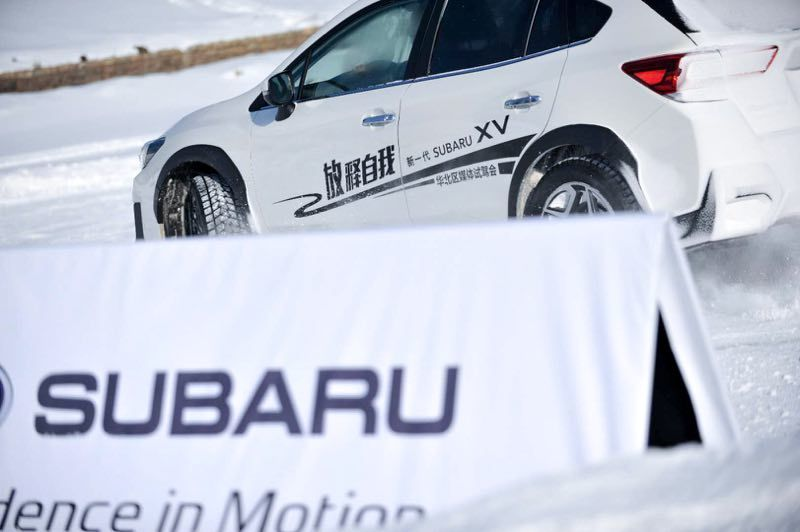尽管我们从外观来看，新一代XV相较老款车型并没有大的变动，更多的不同是体现在细微之处，比如进气格珊、前大灯和尾灯以及轮圈等细节。而整体的造型理念和车身轮廓相较老款车型来说并没有什么变化。还记得新一代XV在发布的时候曾有评论。对于新一代斯巴鲁来说，我们将更多精力投入到了新平台与动力总成的研发和升级工作。于是便诞生了中国市场上首款汇聚水平对置发动机（SUBARU BOXER）、左右对称全时四轮驱动系统（Symmetrical AWD）、斯巴鲁全球化平台（SUBARU GLOBAL PLATFORM）、EyeSight驾驶辅助系统四大核心汽车技术的车型。

动力方面，新一代斯巴鲁XV搭载了代号FB20的2.0开自然吸气发动机。缸内直喷技术的应用和压缩比的提升，使其拥有156马力的最大功率。，峰值扭矩为196牛·米，与之相匹配的是LineartronicCVT无级变速箱，虽然依是CVT，但也有不少进步，首先它的模拟挡位从6挡变成7挡，然后他的齿比范围也从之前的6.28提升高了7.03，有助于提升高速行驶时的燃油经济性。其次，通过缩短链条节距，还一并实现了传动效率的提高和自身振动及噪音的降低。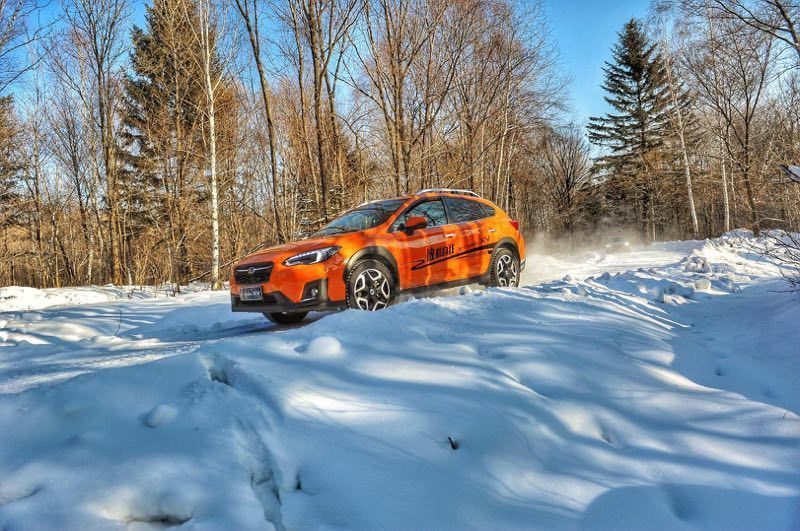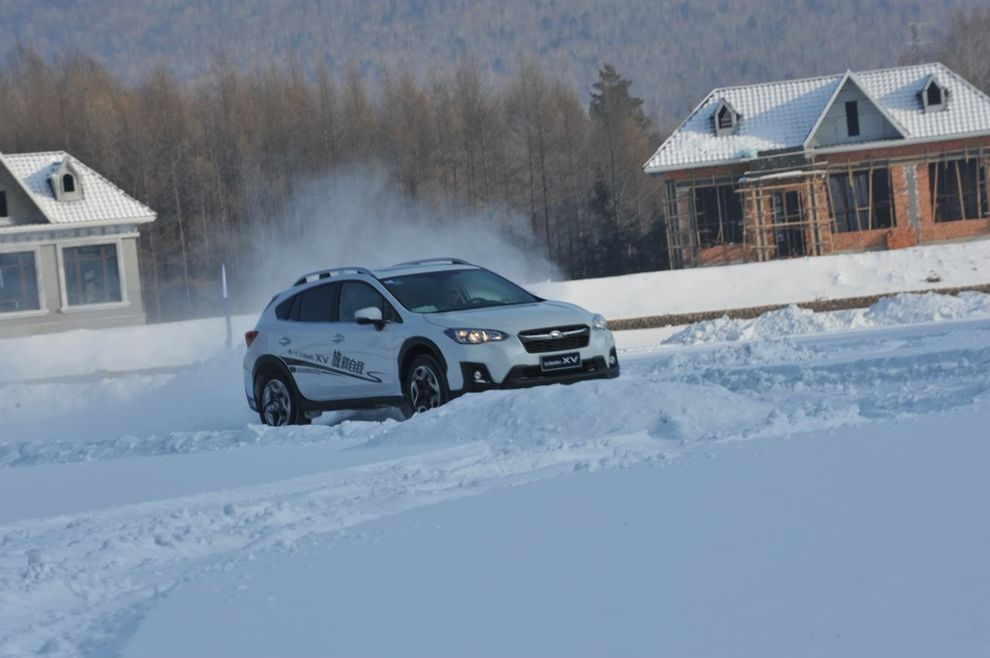本次冰雪试驾体验主要是越野体验以及冰雪场地对比试驾。其中，湖面上设置的专业冰雪场地试驾让新一代SUBARU XV更为直接地秀出性能。我们知道，水平对置发动机与生俱来的低重心和左右对称的全时四驱系统，一直以来都是让斯巴鲁在弯道中自信满满的法宝，新一代XV自不例外。在直线加速体验中，拜由于斯巴鲁全球化平台（SUBARU GLOBAL PLATFORM）应用的加持，提高了车辆的车身、底盘刚性，同时以更为合理的布局进一步降低了重心，加之左右对称全时四轮驱动系统（Symmetrical AWD）带来的完美平衡性能和抓地力，即使在积雪覆盖、开阔侧风较大的冰湖上驾驶，人们仍然能够感受到超常稳定的直线行驶。我们可以明确的感受到，新一代XV的响应显得更为积极，指向清晰、路感指向丰富。另外，紧急制动测试中，斯巴鲁新研发的卡钳和刹车片将制动距离大幅缩短，优化后的助力器也使踩踏感受更加舒适。车辆整体并没有过多的摆动，同时也并未发生侧滑现象，有一种很可靠的感觉。麋鹿测试中，再次降低的车身重心与刚性提升70-100%的车身和底盘，共同造就了精准操控和极强的危险规避能力。而在越野项目体验式，各位媒体老师共同驾车穿越白雪覆盖的山林，由于道路极窄而且路况不明，笔者在体验开始之前不由得有些担心，但是当体验正式开始之时笔者的担心也就烟消云散了。斯巴鲁最新配备的X-MODE使车辆的四驱性能提升约20%，无论坑洼路还是遭遇车轮悬空，新一代SUBARU XV展现出强大的脱困能力和道路适应性都远超人们对一辆城市SUV的期待。同时得益于斯巴鲁XV220mm的高离地间隙，以及接近专业越野车的18度接近角与29度离去角等优势，小编得以顺利通过，在低附着力的积雪坡道，斯巴鲁XV依旧能应付自如。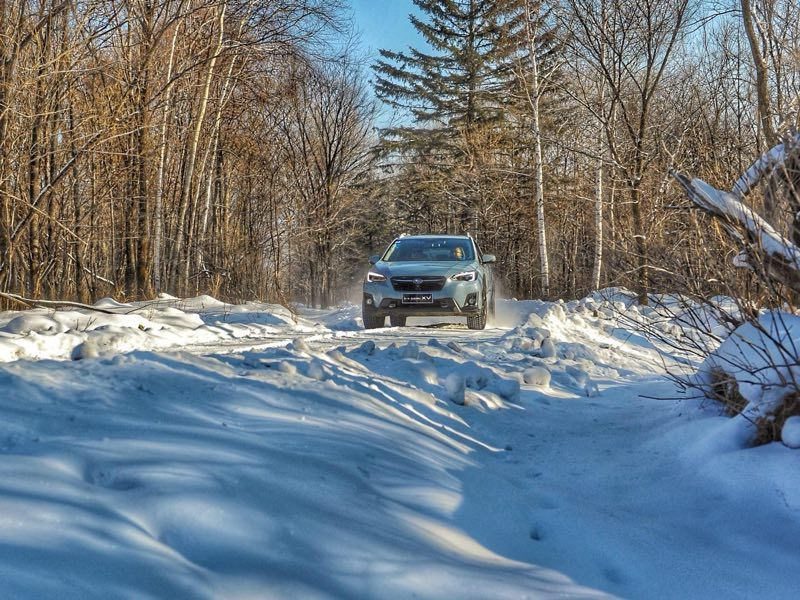在征服所有复杂道路后，参与者们进行了温泉、雪地摩托等冬季休闲项目体验，一行人赏雪景，呼吸凛冽沁脾的新鲜空气，陶醉于美景与这难得的户外运动享受，真是其乐无穷。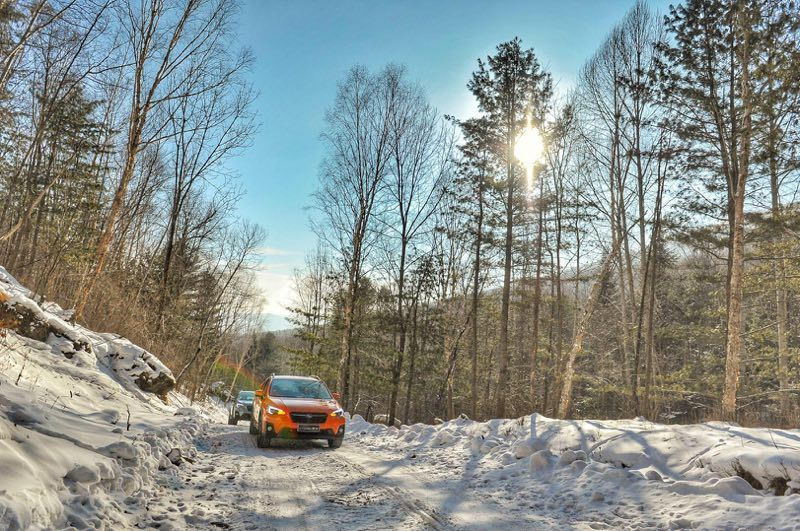结语：虽然此次冰雪试驾只是一个简单的体验，但众多电子安全系统的配备能够让新一代XV在这样一个恶劣的环境下带着我尽情撒野，作为斯巴鲁全新平台的产品，新一代XV在保持驾驶乐趣的同时又兼顾了燃油经济性。相信在不久将来，新一代XV会有优秀的市场表现。

|分享

•已阅！握爪
•我手滑为你点赞
•128个赞！
•不明觉厉
•阅后既醉
•有钱！任性
•照片太美，我不敢看
•干货！杠杠的
•高大上
•膜拜中！
•请接受我的膝盖
•猫哥/猫妹么么哒
•神吐槽
•我只笑笑不说话
•我想静静
•窒息
##### 热度排行• 阿斯顿·马丁
• 奥迪
• 阿尔法罗密欧
• ALPINA

• 巴博斯
• 宝骏
• 宝马
• 保时捷
• 北汽制造
• 奔驰
• 奔腾
• 本田
• 比亚迪
• 标致
• 别克
• 宾利
• 布加迪
• 北汽威旺
• 北京
• 北汽绅宝
• 北汽幻速
• 北汽新能源
• 宝沃
• 比速汽车
• 北汽道达

• 昌河
• 长安
• 长城
• 长安商用
• 成功汽车
• 长江EV
• 长安轻车型

• 大众
• 道奇
• 东风
• 东风风神
• 东风小康
• 东南
• DS
• 东风风行
• 东风风度
• 东风风光

• 法拉利
• 菲亚特
• 丰田
• 福迪
• 福特
• 福田汽车
• 福汽启腾
• 风诺

• GMC
• 广汽传祺
• 广汽吉奥
• 观致

• 哈飞
• 海格
• 海马
• 华泰
• 黄海
• 恒天
• 红旗
• 哈弗
• 华颂
• 华凯
• 华泰新能源
• 汉腾汽车

• Jeep
• 江淮
• 江铃
• 捷豹
• 金杯
• 九龙
• 吉利汽车
• 金旅
• 金龙
• 江铃集团轻汽
• 江铃集团新能源
• 君马汽车
• 捷途

• 开瑞
• 凯迪拉克
• 科尼赛克
• 克莱斯勒
• KTM
• 卡威
• 凯翼
• 康迪
• 康迪电动汽车集团

• 猎豹汽车
• 兰博基尼
• 劳斯莱斯
• 雷克萨斯
• 雷诺
• 理念
• 力帆
• 莲花汽车
• 林肯
• 铃木
• 陆风
• 路虎
• 路特斯
• 领志
• 领克
• 零跑汽车

• MG
• MINI
• 马自达
• 玛莎拉蒂
• 迈凯伦
• 摩根

• 纳智捷

• 讴歌
• 欧宝
• 欧朗
• 欧拉
• 欧尚汽车

• 帕加尼

• 奇瑞
• 启辰
• 起亚
• 前途
• 庆铃汽车

• 日产
• 荣威
• 瑞麒汽车
• 如虎
• 瑞驰

• 上汽大通
• smart
• 三菱
• 双环
• 双龙
• 斯巴鲁
• 斯柯达
• 萨博
• 思铭
• 赛麟
• SWM斯威汽车

• TESLA
• 泰卡特
• 腾势

• 威麟
• 威兹曼
• 沃尔沃
• 五菱汽车
• 五十铃
• 潍柴英致
• WEY
• 蔚来
• 威马汽车

• 现代
• 雪佛兰
• 雪铁龙
• 西雅特
• 新特汽车
• 小鹏汽车
• 新宝骏

• 野马汽车
• 一汽
• 依维柯
• 英菲尼迪
• 永源
• 驭胜

• 中华
• 中兴
• 众泰
• 知豆
• 之诺
• 正道汽车
• A
• B
• C
• D
• E
• F
• G
• H
• I
• J
• K
• L
• M
• N
• O
• P
• Q
• R
• S
• T
• U
• V
• W
• X
• Y
• Z

• 阿斯顿·马丁
• 奥迪
• 阿尔法罗密欧
• ALPINA

• 巴博斯
• 宝骏
• 宝马
• 保时捷
• 北汽制造
• 奔驰
• 奔腾
• 本田
• 比亚迪
• 标致
• 别克
• 宾利
• 布加迪
• 北汽威旺
• 北京
• 北汽绅宝
• 北汽幻速
• 北汽新能源
• 宝沃
• 比速汽车
• 北汽道达

• 昌河
• 长安
• 长城
• 长安商用
• 成功汽车
• 长江EV
• 长安轻车型

• 大众
• 道奇
• 东风
• 东风风神
• 东风小康
• 东南
• DS
• 东风风行
• 东风风度
• 东风风光

• 法拉利
• 菲亚特
• 丰田
• 福迪
• 福特
• 福田汽车
• 福汽启腾
• 风诺

• GMC
• 广汽传祺
• 广汽吉奥
• 观致

• 哈飞
• 海格
• 海马
• 华泰
• 黄海
• 恒天
• 红旗
• 哈弗
• 华颂
• 华凯
• 华泰新能源
• 汉腾汽车

• Jeep
• 江淮
• 江铃
• 捷豹
• 金杯
• 九龙
• 吉利汽车
• 金旅
• 金龙
• 江铃集团轻汽
• 江铃集团新能源
• 君马汽车
• 捷途

• 开瑞
• 凯迪拉克
• 科尼赛克
• 克莱斯勒
• KTM
• 卡威
• 凯翼
• 康迪
• 康迪电动汽车集团

• 猎豹汽车
• 兰博基尼
• 劳斯莱斯
• 雷克萨斯
• 雷诺
• 理念
• 力帆
• 莲花汽车
• 林肯
• 铃木
• 陆风
• 路虎
• 路特斯
• 领志
• 领克
• 零跑汽车

• MG
• MINI
• 马自达
• 玛莎拉蒂
• 迈凯伦
• 摩根

• 纳智捷

• 讴歌
• 欧宝
• 欧朗
• 欧拉
• 欧尚汽车

• 帕加尼

• 奇瑞
• 启辰
• 起亚
• 前途
• 庆铃汽车

• 日产
• 荣威
• 瑞麒汽车
• 如虎
• 瑞驰

• 上汽大通
• smart
• 三菱
• 双环
• 双龙
• 斯巴鲁
• 斯柯达
• 萨博
• 思铭
• 赛麟
• SWM斯威汽车

• TESLA
• 泰卡特
• 腾势

• 威麟
• 威兹曼
• 沃尔沃
• 五菱汽车
• 五十铃
• 潍柴英致
• WEY
• 蔚来
• 威马汽车

• 现代
• 雪佛兰
• 雪铁龙
• 西雅特
• 新特汽车
• 小鹏汽车
• 新宝骏

• 野马汽车
• 一汽
• 依维柯
• 英菲尼迪
• 永源
• 驭胜

• 中华
• 中兴
• 众泰
• 知豆
• 之诺
• 正道汽车
• A
• B
• C
• D
• E
• F
• G
• H
• I
• J
• K
• L
• M
• N
• O
• P
• Q
• R
• S
• T
• U
• V
• W
• X
• Y
• Z

• 阿斯顿·马丁
• 奥迪
• 阿尔法罗密欧
• ALPINA

• 巴博斯
• 宝骏
• 宝马
• 保时捷
• 北汽制造
• 奔驰
• 奔腾
• 本田
• 比亚迪
• 标致
• 别克
• 宾利
• 布加迪
• 北汽威旺
• 北京
• 北汽绅宝
• 北汽幻速
• 北汽新能源
• 宝沃
• 比速汽车
• 北汽道达

• 昌河
• 长安
• 长城
• 长安商用
• 成功汽车
• 长江EV
• 长安轻车型

• 大众
• 道奇
• 东风
• 东风风神
• 东风小康
• 东南
• DS
• 东风风行
• 东风风度
• 东风风光

• 法拉利
• 菲亚特
• 丰田
• 福迪
• 福特
• 福田汽车
• 福汽启腾
• 风诺

• GMC
• 广汽传祺
• 广汽吉奥
• 观致

• 哈飞
• 海格
• 海马
• 华泰
• 黄海
• 恒天
• 红旗
• 哈弗
• 华颂
• 华凯
• 华泰新能源
• 汉腾汽车

• Jeep
• 江淮
• 江铃
• 捷豹
• 金杯
• 九龙
• 吉利汽车
• 金旅
• 金龙
• 江铃集团轻汽
• 江铃集团新能源
• 君马汽车
• 捷途

• 开瑞
• 凯迪拉克
• 科尼赛克
• 克莱斯勒
• KTM
• 卡威
• 凯翼
• 康迪
• 康迪电动汽车集团

• 猎豹汽车
• 兰博基尼
• 劳斯莱斯
• 雷克萨斯
• 雷诺
• 理念
• 力帆
• 莲花汽车
• 林肯
• 铃木
• 陆风
• 路虎
• 路特斯
• 领志
• 领克
• 零跑汽车

• MG
• MINI
• 马自达
• 玛莎拉蒂
• 迈凯伦
• 摩根

• 纳智捷

• 讴歌
• 欧宝
• 欧朗
• 欧拉
• 欧尚汽车

• 帕加尼

• 奇瑞
• 启辰
• 起亚
• 前途
• 庆铃汽车

• 日产
• 荣威
• 瑞麒汽车
• 如虎
• 瑞驰

• 上汽大通
• smart
• 三菱
• 双环
• 双龙
• 斯巴鲁
• 斯柯达
• 萨博
• 思铭
• 赛麟
• SWM斯威汽车

• TESLA
• 泰卡特
• 腾势

• 威麟
• 威兹曼
• 沃尔沃
• 五菱汽车
• 五十铃
• 潍柴英致
• WEY
• 蔚来
• 威马汽车

• 现代
• 雪佛兰
• 雪铁龙
• 西雅特
• 新特汽车
• 小鹏汽车
• 新宝骏

• 野马汽车
• 一汽
• 依维柯
• 英菲尼迪
• 永源
• 驭胜

• 中华
• 中兴
• 众泰
• 知豆
• 之诺
• 正道汽车
• A
• B
• C
• D
• E
• F
• G
• H
• I
• J
• K
• L
• M
• N
• O
• P
• Q
• R
• S
• T
• U
• V
• W
• X
• Y
• Z